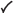# prob2struct

## 语法

``problem = prob2struct(prob)``
``problem = prob2struct(prob,x0)``
``problem = prob2struct(___,Name,Value)``

## 说明

``problem = prob2struct(prob)` 返回适合基于求解器求解的优化问题结构体。对于非线性问题，`prob2struct` 会为目标函数创建文件，如有必要，还为非线性约束函数和支持文件创建文件。`

``problem = prob2struct(prob,x0)` 还转换初始点结构体 `x0`，并将其包含在 `problem` 中。`

``problem = prob2struct(___,Name,Value)` 可针对任何输入参数使用一个或多个名称-值对组参数指定附加选项。例如，对于非线性优化问题，`problem = prob2struct(prob,'ObjectiveFunctionName','objfun1')` 指定 `prob2struct` 在当前文件夹中创建一个名为 `objfun1.m` 的目标函数文件。`

## 示例

```ingots = optimvar('ingots',4,1,'Type','integer','LowerBound',0,'UpperBound',1); alloys = optimvar('alloys',4,1,'LowerBound',0); weightIngots = [5,3,4,6]; costIngots = weightIngots.*[350,330,310,280]; costAlloys = [500,450,400,100]; cost = costIngots*ingots + costAlloys*alloys; steelprob = optimproblem; steelprob.Objective = cost; totalweight = weightIngots*ingots + sum(alloys); carbonIngots = [5,4,5,3]/100; molybIngots = [3,3,4,4,]/100; carbonAlloys = [8,7,6,3]/100; molybAlloys = [6,7,8,9]/100; totalCarbon = (weightIngots.*carbonIngots)*ingots + carbonAlloys*alloys; totalMolyb = (weightIngots.*molybIngots)*ingots + molybAlloys*alloys; steelprob.Constraints.conswt = totalweight == 25; steelprob.Constraints.conscarb = totalCarbon == 1.25; steelprob.Constraints.consmolyb = totalMolyb == 1.25;```

`problem = prob2struct(steelprob);`

`Aeq = problem.Aeq`
```Aeq = (1,1) 1.0000 (2,1) 0.0800 (3,1) 0.0600 (1,2) 1.0000 (2,2) 0.0700 (3,2) 0.0700 (1,3) 1.0000 (2,3) 0.0600 (3,3) 0.0800 (1,4) 1.0000 (2,4) 0.0300 (3,4) 0.0900 (1,5) 5.0000 (2,5) 0.2500 (3,5) 0.1500 (1,6) 3.0000 (2,6) 0.1200 (3,6) 0.0900 (1,7) 4.0000 (2,7) 0.2000 (3,7) 0.1600 (1,8) 6.0000 (2,8) 0.1800 (3,8) 0.2400 ```
`beq = problem.beq`
```beq = 3×1 25.0000 1.2500 1.2500 ```

`problem.lb`
```ans = 8×1 0 0 0 0 0 0 0 0 ```
`problem.ub`
```ans = 8×1 Inf Inf Inf Inf 1 1 1 1 ```

`x = intlinprog(problem)`
```LP: Optimal objective value is 8125.600000. Cut Generation: Applied 3 mir cuts. Lower bound is 8495.000000. Relative gap is 0.00%. Optimal solution found. Intlinprog stopped at the root node because the objective value is within a gap tolerance of the optimal value, options.AbsoluteGapTolerance = 0 (the default value). The intcon variables are integer within tolerance, options.IntegerTolerance = 1e-05 (the default value). ```
```x = 8×1 7.2500 0 0.2500 3.5000 1.0000 1.0000 0 1.0000 ```

```x = optimvar('x',2); fun = 100*(x(2) - x(1)^2)^2 + (1 - x(1))^2; prob = optimproblem('Objective',fun); mycon = dot(x,x) <= 4; prob.Constraints.mycon = mycon; x0.x = [-1;1.5];```

`prob` 转换为优化问题结构体。将生成的目标函数文件命名为 `'rosenbrock'`，将约束函数文件命名为 `'circle2'`

```problem = prob2struct(prob,x0,'ObjectiveFunctionName','rosenbrock',... 'ConstraintFunctionName','circle2');```

`prob2struct` 在当前文件夹中创建非线性目标和约束函数文件。要在一个不同文件夹中创建这些文件，请使用 `'FileLocation'` 名称-值对组。

`[x,fval] = fmincon(problem)`
```Local minimum found that satisfies the constraints. Optimization completed because the objective function is non-decreasing in feasible directions, to within the value of the optimality tolerance, and constraints are satisfied to within the value of the constraint tolerance. ```
```x = 2×1 1.0000 1.0000 ```
```fval = 4.6187e-11 ```

## 输入参数

### 名称-值参数

```options = optimoptions('fmincon','SpecifyObjectiveGradient',true,... 'SpecifyConstraintGradient',true); problem.options = options;```

`prob2struct` 为优化问题创建的非线性约束函数文件的名称，指定为由 `'ConstraintFunctionName'` 和文件名组成的以逗号分隔的对组。此参数适用于 `fmincon``fminunc` 问题；请参阅 `problem`。文件名中不要包含文件扩展名 `.m``prob2struct` 会在创建文件时追加文件扩展名。

`prob2struct` 为方程问题创建的非线性方程函数文件的名称，指定为由 `'EquationFunctionName'` 和文件名组成的以逗号分隔的对组。此参数适用于 `fsolve``fzero``lsqnonlin` 方程；请参阅`problem`。文件名中不要包含文件扩展名 `.m``prob2struct` 会在创建文件时追加文件扩展名。

```options = optimoptions('fmincon','SpecifyObjectiveGradient',true,... 'SpecifyConstraintGradient',true); problem.options = options;```

`prob2struct` 为优化问题创建的目标函数文件的名称，指定为由 `'ObjectiveFunctionName'` 和文件名组成的以逗号分隔的对组。此参数适用于 `fmincon``fminunc` 问题；请参阅 `problem`。文件名中不要包含文件扩展名 `.m``prob2struct` 会在创建文件时追加文件扩展名。

`fminunc` 用于没有约束的问题，否则使用 `fmincon`

LPMILPQPSOCP线性最小二乘非线性最小二乘NLPMINLP

`linprog`xxxxxxx
`intlinprog`xxxxxx
`quadprog`xxxx
`coneprog`xxxxxx
`lsqlin`xxxxxxx
`lsqnonneg`xxxxxxx
`lsqnonlin`xxxxxx
`fminunc`xxx
`fmincon`xx
`patternsearch` (Global Optimization Toolbox)xx
`ga` (Global Optimization Toolbox)`particleswarm` (Global Optimization Toolbox)xxx
`simulannealbnd` (Global Optimization Toolbox)xxx
`surrogateopt` (Global Optimization Toolbox)`gamultiobj` (Global Optimization Toolbox)`paretosearch` (Global Optimization Toolbox)xx

• * 表示该问题类型的默认求解器。

• Y 表示可用的求解器。

• N 表示不可用的求解器。

## 输出参数

`intlinprog`

`linprog`

`lsqlin`

`lsqnonlin`

`quadprog`

`fminunc`

`fmincon`

`ga`

`lsqlin`

`fzero`

`fsolve`

`lsqnonlin`

## 提示

• 如果您为非线性问题在同一 MATLAB® 会话中多次调用 `prob2struct`，请使用 `ObjectiveFunctionName``EquationFunctionName` 参数，如果合适，还使用 `ConstraintFunctionName` 参数。指定唯一名称可以确保生成的问题结构体引用正确的目标和约束函数。否则，对 `prob2struct` 的后续调用可能导致生成的非线性函数文件覆盖现有文件。

• 为了避免导致无限递归，请不要在目标或约束函数内调用 `prob2struct`

• 当为非线性问题并行调用 `prob2struct` 时，请确保生成的目标和约束函数文件具有唯一名称。这样做可以避免每次循环遍历写入相同的一个或多个文件。

## 算法

### 转换为求解器形式

• `x` - 3×2×4 数组

• `y` - 3×2 数组

`vars` 的前 24 个元素等效于 `x(:)`，接下来的 6 个元素等效于 `y(:)`，总共 30 个元素。下界和上界对应于此变量顺序，每个线性约束矩阵有 30 列。

### 自动微分

• 对于一般的非线性目标函数，`fmincon` 默认选择反向 AD。对于非线性约束函数，如果其非线性约束的数量小于变量数目，`fmincon` 默认选择反向 AD。否则，`fmincon` 默认选择正向 AD。

• 对于一般的非线性目标函数，`fminunc` 默认选择反向 AD。

• 对于最小二乘目标函数，`fmincon``fminunc` 对目标函数默认选择正向 AD。有关基于问题的最小二乘目标函数的定义，请参阅Write Objective Function for Problem-Based Least Squares

• 当目标向量中的元素数大于或等于变量数时，`lsqnonlin` 默认选择正向 AD。否则，`lsqnonlin` 默认选择反向 AD。

• 当方程数大于或等于变量数时，`fsolve` 默认选择正向 AD。否则，`fsolve` 默认选择反向 AD。

```options = optimoptions('fmincon','SpecifyObjectiveGradient',true,... 'SpecifyConstraintGradient',true); problem.options = options;```